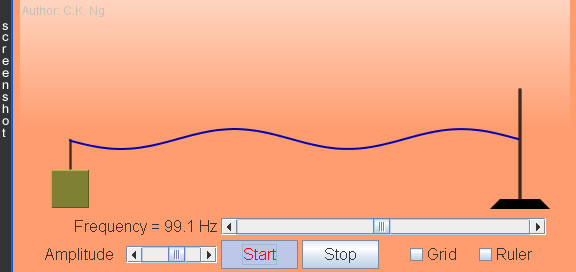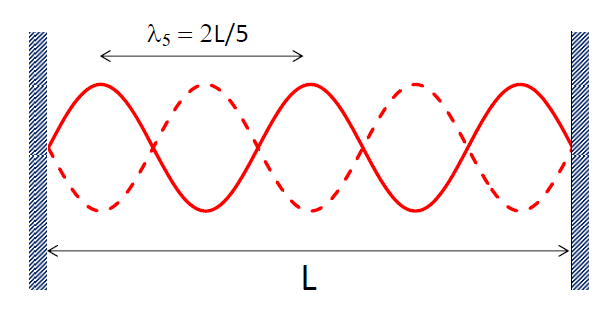Generating Standing Waves in String (Resonance)

The length of the string can be varied by dragging the stand to the left/right.
The tension in the string can hence be changed.

 Internal Links : Mechanical Resonance Electrical Resonance I Electrical Resonance II

• A stationary wave is produced when the wavelength of the wave in the string being fixed at its two ends satisfies λn = 2L/n, where L is the length of the string and n = 1, 2, 3,…
• When a stationary wave of wavelength λn is formed, there are n loops formed in the string.• Each stationary wave is a normal mode of the system.
• Besides the normal modes, the string has infinite modes of oscillations, e.g., plucking the string at any point on it. However, any mode can be represented by a series sum of the normal modes of different amplitudes. An analogy in mathematics is that any 3D vector can be expressed like P = a i + b j+ c k, where ij and k are the unit vectors.
• The system is forced to oscillate when a periodic force is applied to it. When the frequency of the periodic force matches with one of the frequencies fn = wave speed / λn, resonance will occur. Then, stationary wave of that normal mode appears on the string.
• When the system is forced to oscillate at off-resonance, the oscillation is a combination of the neighboring modes. The resultant amplitude is usually small becuse none of the normal modes is dominant.

 The author (Chiu-king Ng) has the copyright on all the simulations in this website. Email phyAA@phy.hk, where AA is the prime number following 7. Last Update:2019-3-20